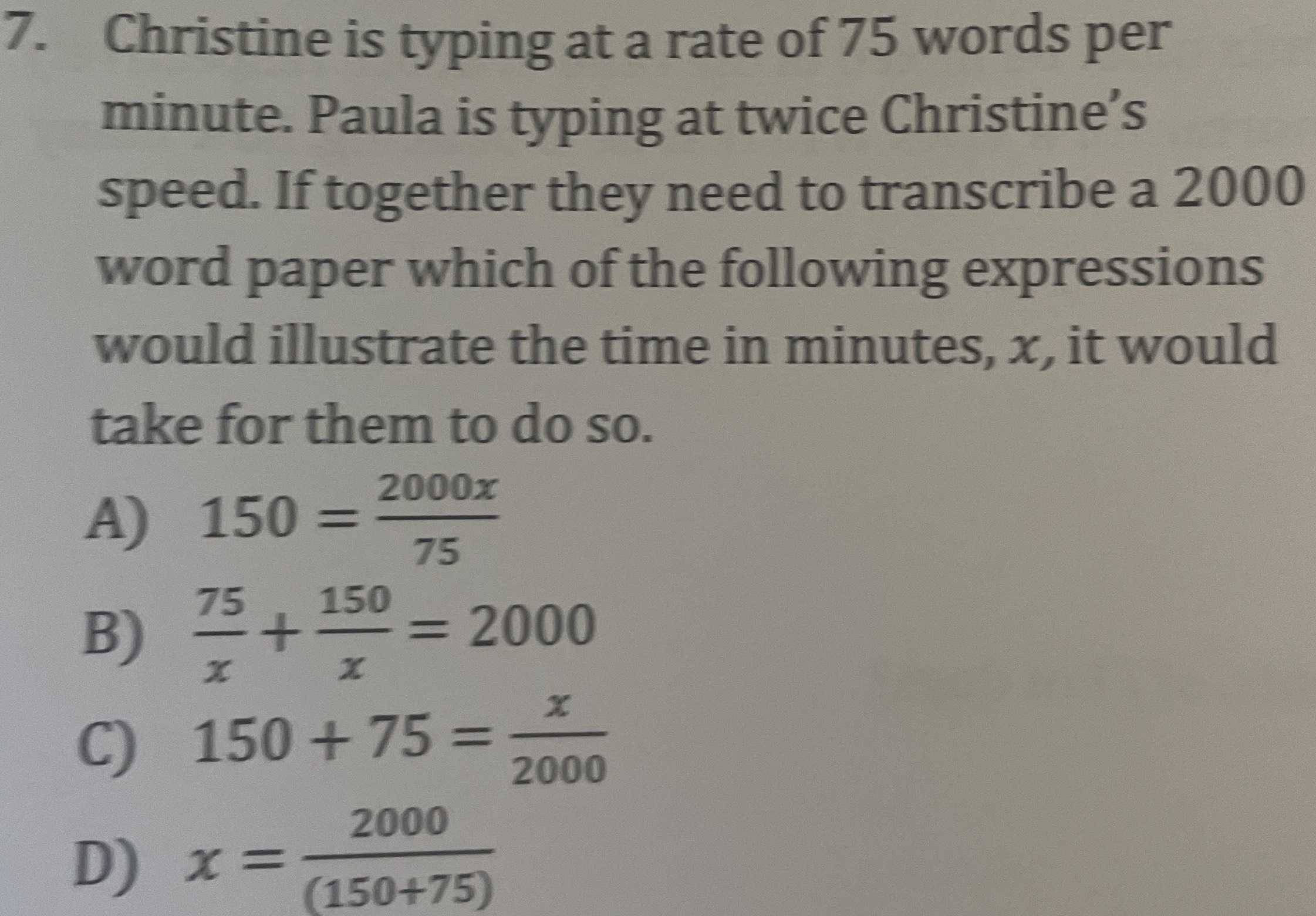### Still have math questions?

Algebra
QuestionChristine is typing at a rate of $$75$$ words per minute. Paula is typing at twice Christine's speed. If together they need to transcribe a $$2000$$ word paper which of the following expressions would illustrate the time in minutes, $$x$$ , it would take for them to do so.

A) $$150 = \frac { 2000 x } { 75 }$$

B) $$\frac { 75 } { x } + \frac { 150 } { x } = 2000$$

C) $$150 + 75 = \frac { x } { 2000 }$$

D) $$x = \frac { 2000 } { ( 150 + 75 ) }$$